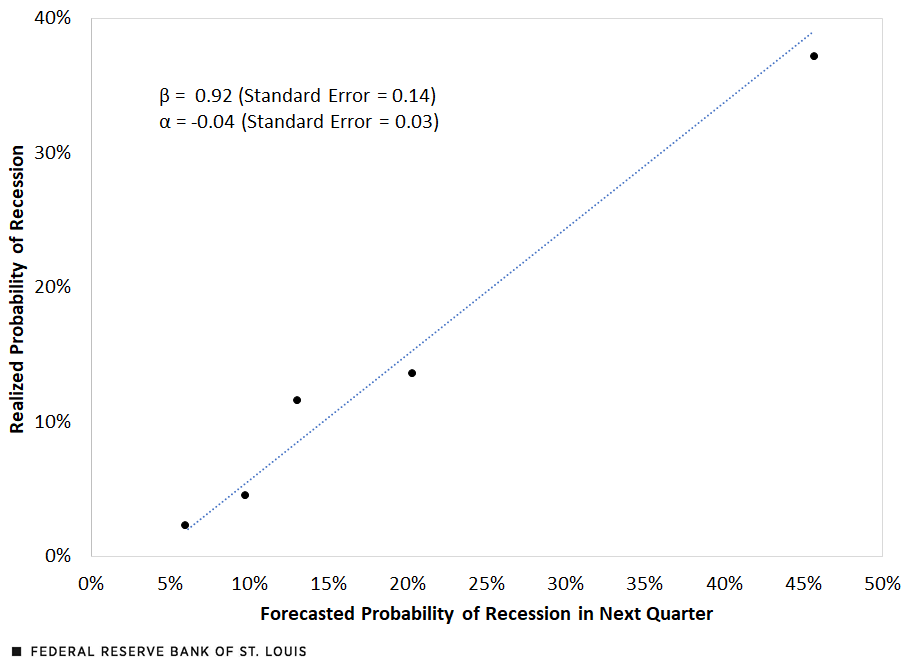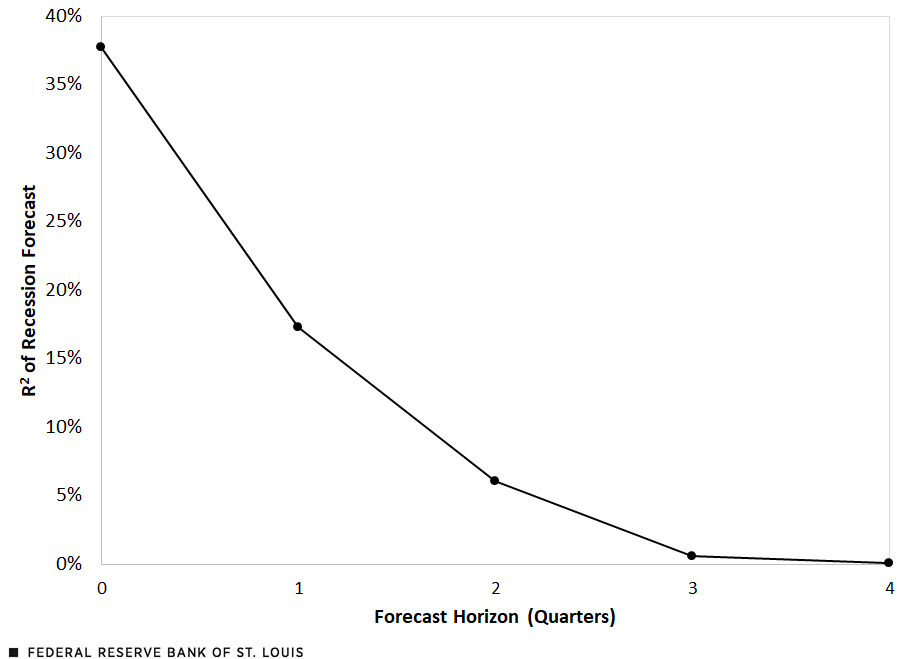# Can Economists Predict Recessions?

September 26, 2023

When people ask questions about the economy, they often ask about predictions. Will there be a recession soon? When will inflation come down? Why didn’t economists see the 2008 crisis coming? And perhaps most importantly: Do economists have any idea what’s going to happen to the economy?

These questions have taken on particular importance recently. In the past four quarters, economic forecasters have, on average, predicted a 42% probability of a contraction in the U.S. economy in the next quarter, according to the Survey of Professional Forecasters (SPF) conducted by the Federal Reserve Bank of Philadelphia. So far, no recession has occurred, although the forecasted risk of a downturn in the fourth quarter remains elevated at 34.4%.

At the same time, policymakers, including those at the Federal Reserve, rely on economic forecasts (both external, such as the SPF, and internal, such as the Fed Board of Governors’ Tealbook forecasts) to inform how they should adjust interest rates. If the economy is expected to remain hot (high inflation and low unemployment), that will typically call for higher interest rates than if the economy is expected to cool (falling inflation and weaker economic growth).

## Accuracy vs. Precision in Economic Forecasting

How well can economists predict recessions? To answer this question, we need to distinguish between two properties of a good forecast: accuracy and precision. A forecast is accurate if it is, on average, correct. So, for example, if the weather forecast says that there is a 30% chance of rain tomorrow, we would say the forecast is accurate if, on average, it rains 30% of the time the following day.

A precise forecast leaves less uncertainty about what will happen in the future. A forecast that says there is a 50% chance of rain Monday and a 50% chance of rain Tuesday is less precise than a forecast that gives a 99% chance of rain Monday and a 1% chance of rain Tuesday.

A forecast can be accurate without being precise. For example, month-ahead weather forecasts are typically not very precise: The Weather Channel currently predicts a high of 63 degrees on Halloween in St. Louis, but this is just a statistical average for that time of year. Precise forecasts leave less uncertainty than imprecise forecasts.

## Are Economic Forecasts Accurate?

Let’s first examine whether economic forecasts are accurate. We’ll focus on quarterly recession probability forecasts from the SPF. These are projections by private economic forecasters estimating the probability that growth in real gross domestic product (GDP) will be negative in a given quarter. We can compare the recession probabilities provided by the forecasters (averaging across respondents) to what actually happened (whether real GDP growth was actually negative that quarter).

If forecasts are accurate, then when forecasters predict a 15% probability of a recession next quarter, there will, in fact, be a recession 15% of the time. We can summarize the performance of the recession forecasts with a linear regression:

A recession indicator at time t is equal to the constant term plus the slope times the forecasted recession probability at time t plus the error term at time t.

Here, we regress an indicator for whether a recession occurred Recession subscript t on the average recession probability provided by the forecasters Recession underscore Probability subscript t. The constant term, α, reflects the probability of a recession actually occurring if the forecasters predict there is zero chance of recession. If the forecasts are accurate, α should be 0. The slope term, β, reflects how the actual probability of a recession changes as the forecasted probability of a recession changes. If the forecasts are accurate, β should be 1. (epsilon subscript t is the error term.)

In the figure below, we compare the actual probability of a recession, plotted on the vertical axis, with the one-quarter-ahead mean predicted probability of a recession from the SPF, using data from the fourth quarter of 1968 to the third quarter of 2023.

The Relationship between Forecasted and Realized Probabilities of RecessionSOURCES: Philadelphia Fed’s Survey of Professional Forecasters, Bureau of Economic Analysis and author’s calculations.

The five dots represent quintile bins: We sort the data into five quintiles by predicted recession probability, and each dot corresponds to the average predicted probability and actual probability of a recession within that quintile. The dotted line is the best-fit line corresponding to the linear regression described above. The regression finds a slope of 0.92 and an intercept of -0.04, and a slope and intercept of 1 and 0, respectively, are inside the 95% confidence interval. This suggests that the quarter-ahead recession forecasts are fairly accurate.

## Determining the Precision of Forecasts

Let’s now examine how precise economic forecasts are. Can quarter-ahead recession forecasts predict much of the variation in whether a recession actually occurs? A common way to measure a variable’s explanatory power is the R squared: How much of the variance of the outcome (whether a recession actually occurs) is explained by forecasters’ predicted probability of a recession? Based on our earlier linear regression, the R squared is 17%.This estimate comes from our linear regression and does not rely on the assumption that forecasts are accurate. If we assume that forecasts are accurate, then in this case, we can also compute the R squared based only on the forecasts, using the formula the multiple of the inverse of N times the summation of p subscript t times 1 minus p subscript t, which is then divided by the multiple of p bar times 1 minus p bar, where pt is the forecasted recession probability in quarter t, and p bar is the average recession probability across all quarters. This yields the estimate 16%, which is very similar to our regression-based estimate. This suggests that economic forecasts are helpful for predicting the probability of recession, but there is still a great deal of uncertainty; most of the variance is not seen a quarter ahead.

The precision of forecasts also depends on how far out the forecast is made. In the figure below, we plot the recession forecast R squared at different horizons: from zero quarters ahead (i.e., a nowcast) to four quarters ahead.

The Precision of Recession ForecastsSOURCES: Philadelphia Fed’s Survey of Professional Forecasters, Bureau of Economic Analysis and author’s calculations.

The forecast precision falls dramatically, from an R squared of 38% for the nowcast, to just 0.1% for the four-quarters-ahead forecast. This drop in forecast precision makes sense: The near future is more predictable than the distant future.

Economic forecasters provide useful information about the future state of the economy. But making predictions is hard, especially about the (distant) future. Even though forecasts can help, we must live with significant uncertainty about future economic conditions.

Note

1. This estimate comes from our linear regression and does not rely on the assumption that forecasts are accurate. If we assume that forecasts are accurate, then in this case, we can also compute the R squared based only on the forecasts, using the formula the multiple of the inverse of N times the summation of p subscript t times 1 minus p subscript t, which is then divided by the multiple of p bar times 1 minus p bar, where pt is the forecasted recession probability in quarter t, and p bar is the average recession probability across all quarters. This yields the estimate 16%, which is very similar to our regression-based estimate.Jeremy Majerovitz

Jeremy Majerovitz is an associate economist at the Federal Reserve Bank of St. Louis. Learn more about the author.Jeremy Majerovitz

Jeremy Majerovitz is an associate economist at the Federal Reserve Bank of St. Louis. Learn more about the author.

Related Topics

This blog offers commentary, analysis and data from our economists and experts. Views expressed are not necessarily those of the St. Louis Fed or Federal Reserve System.

Email Us

Media questions

All other blog-related questions